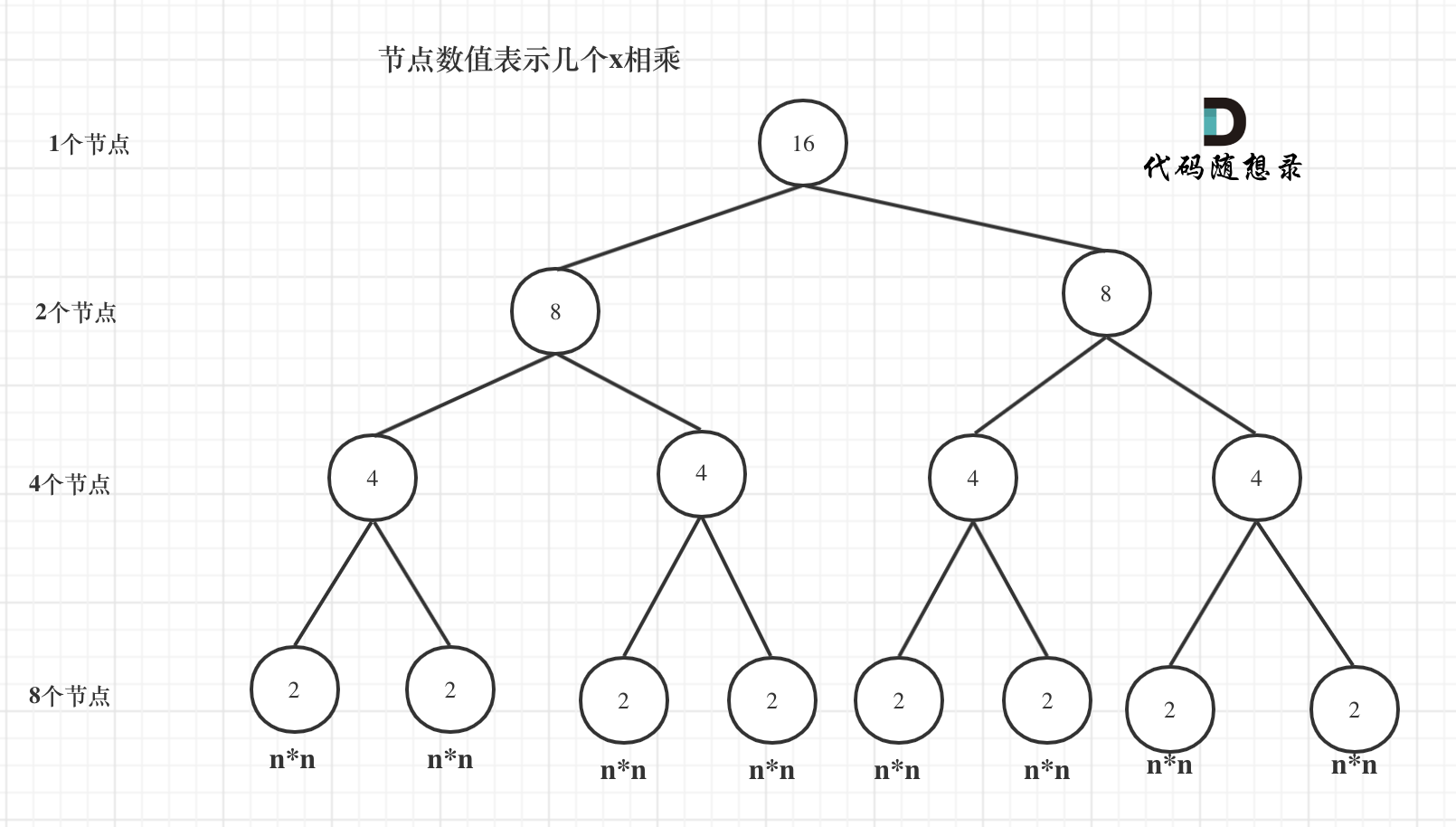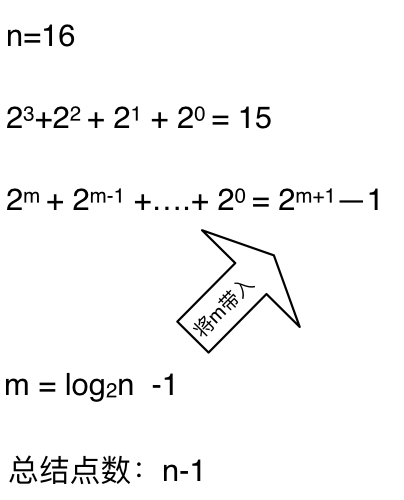# 通过一道面试题目，讲一讲递归算法的时间复杂度！

int function1(int x, int n) {
int result = 1;  // 注意 任何数的0次方等于1
for (int i = 0; i < n; i++) {
result = result * x;
}
return result;
}
1
2
3
4
5
6
7

int function2(int x, int n) {
if (n == 0) {
return 1; // return 1 同样是因为0次方是等于1的
}
return function2(x, n - 1) * x;
}
1
2
3
4
5
6

int function3(int x, int n) {
if (n == 0) {
return 1;
}
if (n % 2 == 1) {
return function3(x, n / 2) * function3(x, n / 2)*x;
}
return function3(x, n / 2) * function3(x, n / 2);
}

1
2
3
4
5
6
7
8
9
10int function4(int x, int n) {
if (n == 0) {
return 1;
}
int t = function4(x, n / 2);// 这里相对于function3，是把这个递归操作抽取出来
if (n % 2 == 1) {
return t * t * x;
}
return t * t;
}
1
2
3
4
5
6
7
8
9
10

# 总结

int function3(int x, int n) {
if (n == 0) {
return 1;
}
if (n % 2 == 1) {
return function3(x, n / 2) * function3(x, n / 2)*x;
}
return function3(x, n / 2) * function3(x, n / 2);
}
1
2
3
4
5
6
7
8
9

@2021-2022 代码随想录 版权所有 粤ICP备19156078号-3